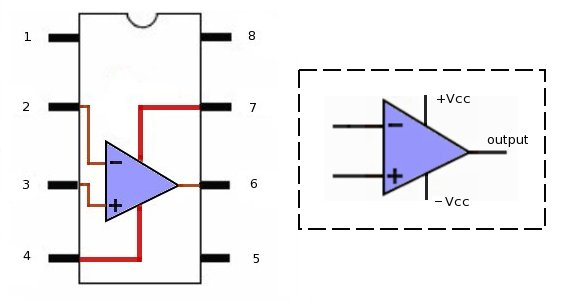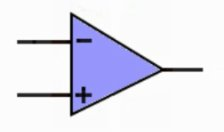black nike shoes roshe cheapnicesports.com

# 741 Op Amp

Operational amplifier or Op-Amp is an analog component, this component was used in old analog computers for performing operations such as addition/multiplication by constant, integration and differentiation etc.. Because of its versatility and reliability, it is very much being used in large number of circuits and devices. Here I won't be discussing about various application of Op-Amp, but rather try to explain Op-Amp a component and the concept which makes this component so special. I hope this article will give you enough food for thought and will enable you to think various applications where the op-amp can be used.

First lets see how OpAmp looks like...

I called op-amp as a component, but it comes as an ICs, so doesn't have its own look like transistors and resistors..Opamp in circuits are represented by much simpler symbol shown below..Opamp is generally represented a three pin device, two input and one output. The power pins +Vcc and -Vcc are normally ignored in diagrams. Now lets see some properties of this Opamp..

1. Opamp has got two input pins (as shown), One with +ve sign is called non-inverting input, while other one with -ve sign is called inverting input.
2. Inputs are called differential input, because difference in voltage levels on these input causes output voltage.
3. What is amplifier in Operational amplifier ? Yes! the output is not just difference between voltage on both input, but it is the voltage difference multiplied by gain.
4. Gain of an OpAmp is generally in range of 106 (very high)
5. Does this means that 1V differential voltage will cause 1000000 Volts at output ? theoretically yes, but practically NO!
Voltage swing of an opamp is limited by its supply voltage (-Vcc to +Vcc only).
6. This high gain is actual strength of opamp which is tamed and used in many applications.
7. If voltage on -ve pin is more than +ve pin, opamp will swing toward -Vcc. And if voltage on +ve pin is more than voltage on -ve pin, the opamp will swing toward +Vcc. e.g if +1v is applied on +ve pin and +2 volts is applied on -ve pin, the opamp will swing toward -Vcc (difference x gain concept).

Again, if +2V is applied on +ve pin and +1V is applied on -ve pin, the opamp will swing toward +Vcc (same, difference x gain concept)
Here we can derive one equation, output_voltage =
[voltage_on_+ve_pin - voltage_on_-ve_pin] * gain

Remember that the output voltage is limited by supply voltage (+Vcc and -Vcc). This means that even though due to high gain opamp may try to shoot at very high output voltage (106), but it will be limited by supply voltage +Vcc and -Vcc.

8. Input Impedance of Opamp is also very high, in range of MegaOhms. This high impedance make it a pure voltage toy, voltage at input, voltage at output simple!

In the next section we will put opamp in various scenarios and will try to analyze its behavior.

But china makes nikes. fake? Its it the eye if the beholder